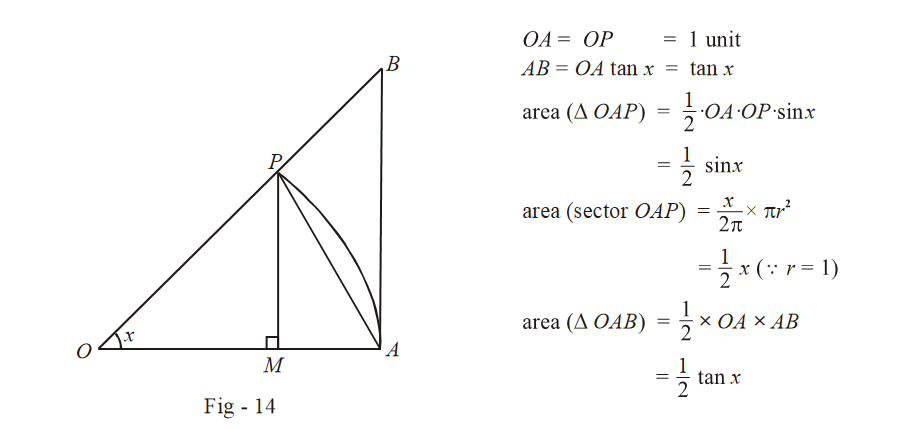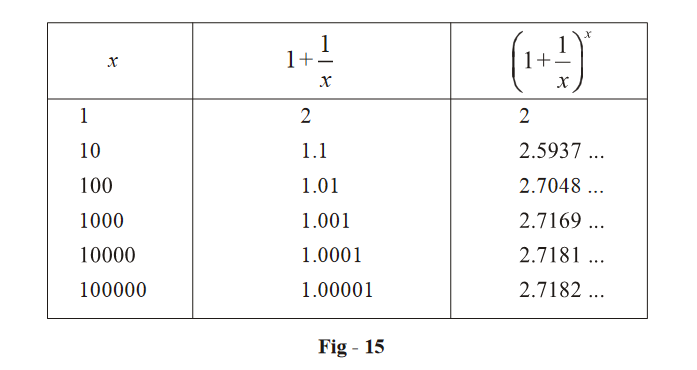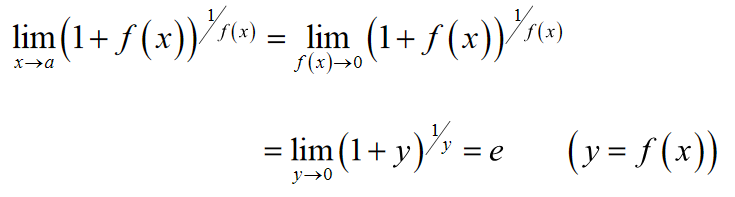# Some Standard Limits

## SOME STANDARD LIMITS:

(A) \begin{align}\fbox{{\mathop {\lim }\limits_{x \to 0} \frac{{\sin x}}{x} = 1;\mathop {\,\,\lim }\limits_{x \to 0} \frac{{\tan x}}{x} = 1}}\end{align}

Both the limits above are indeterminate, of the form \begin{align}\frac{0}{0}\end{align}. We are discussing here a geometric interpretation of these limits. Consider a sector OAP of a unit circle as shown in the figure below.We see that

$${\text{area}}\left( {\Delta\;OAP\;} \right) < {\text{area}}\left( {{\text{sector}}\,\,OAP\;} \right) < {\text{area}}\left( {\Delta OAB} \right)$$

or

\begin{align}\frac{1}{2}\sin x\,\, < \,\,\frac{1}{2}x\,\, < \,\frac{1}{2}\;\tan x\end{align}

or

\begin{align}\frac{{\sin x}}{x}\, < \,\;1\;\, < \,\,\frac{{\tan x}}{x}\end{align}

What happens as x decreases or as $$x \to 0$$?

We see that the difference between the three areas considered above tends to decrease;

$${\text{area}}\left( {\Delta OAP} \right) \to {\text{area}}\left( {{\text{sector}}\,OAP} \right) \leftarrow {\text{area}}\left( {\Delta OAB} \right)$$

$$\Rightarrow \qquad \sin x \to x\;\;\;{\text{and}}\;\;x \to \tan x$$

\Rightarrow \begin{align}\frac{{\sin x}}{x} \to 1\end{align} (but remains less than 1)

\begin{align}\frac{{\tan x}}{x} \to 1\end{align} (remains greater than 1)

$$\Rightarrow$$      $$\fbox{{\mathop {\lim }\limits_{x \to 0} \frac{{\sin x}}{x} = {1^ - } \qquad ;\,\,\,\,\,\,\mathop {\lim }\limits_{x \to 0} \frac{{\tan x}}{x} = {1^ + }}}$$

It is important to observe how any function approaches a limit. For example, in the case above, as $$x \to 0$$ , \begin{align}\frac{{\sin x}}{x}\end{align} approaches 1 from the left side while \begin{align}\frac{{\tan x}}{x}\end{align} approaches 1 from the right side. This makes a big difference. Why?

Consider the limits below and you should understand:

$\mathop {\lim }\limits_{x \to 0} \left[ {\frac{{\sin x}}{x}} \right] = 0\,\,\,\,\,;\,\,\,\,\,\mathop {\lim }\limits_{x \to 0} \left[ {\frac{{\tan x}}{x}} \right] = 1$

(B) $$\fbox{{\mathop {\lim }\limits_{x \to \infty } {{\left( {1 + \frac{1}{x}} \right)}^x} = e}}$$

Consider the expression for $$f\left( x \right) = {\left( {1 + \frac{1}{x}} \right)^x}$$. As x gets larger and larger or as $$x \to \infty$$ , the base \begin{align}\left( {1 + \frac{1}{x}} \right)\end{align} gets closer to 1 while the exponent (x), tends to infinity. Hence, this limit is of the indeterminate form $${1^\infty }$$. Its very important to get a ‘feel’ that the value \begin{align}{\left( {1 + \frac{1}{x}} \right)^x}\end{align} will converge to a fixed, definite value, as x increases. You should get this feel by looking at the table below.We see that as x becomes larger, the term \begin{align}{\left( {1 + \frac{1}{x}} \right)^x}\end{align} converges to some value (This can be proved) This limiting value is denoted by e. e is an irrational number and its value is e = 2.7182... Try to show that the limit of \begin{align}{\left( {1 + \frac{1}{x}} \right)^x}\end{align} is bounded and lies between and 2 and 3, that is, $$2 < e < 3{\rm{ }}$$ you can use the binomial theorem in conjunction with the sandwich theorem to prove this, by first proving it for an integral x)

The limit we have just seen is extremely important and will be widely used subsequently.

Note another important point:

If $$f\left( x \right) \to 0\;\;{\rm{as}}\;\;x \to a,\;\;{\rm{then}}$$Hence, any limit of this form has the value e.

(C)  \begin{align}\fbox{{\mathop {\lim }\limits_{x \to 0} \frac{{\ln \left( {1 + x} \right)}}{x} = 1}}\end{align} $$\left( {\ln \,\,y \equiv {{\log }_e}y} \right)$$

This limit is of the indeterminate form $$\frac{0}{0}$$. We can easily evaluate this limit based on the previous limit.

\begin{align}&\mathop {\lim }\limits_{x \to 0} \frac{{\ln \left( {1 + x} \right)}}{x}\\\\& = \mathop {\lim }\limits_{x \to 0} \frac{1}{x}\ln \left( {1 + x} \right)\\\\& = \mathop {\lim }\limits_{x \to 0} \ln {\left( {1 + x} \right)^{\frac{1}{x}}}\qquad \left( \text{Property of log} \right)\\\\& = \ln \left\{ {\mathop {\lim }\limits_{x \to 0} {{\left( {1 + x} \right)}^{1/x}}} \right\}\\\\ &= \ln \,\,e = \,\,{\log _e}e\,\, = \,\,1\end{align}

This limit can alternatively be evaluated by using the expansion series for ln (1 + x)

$\ln\;\left( {1 + x} \right) = x - \frac{{\,{x^2}}}{2} + \frac{{\,{x^3}}}{3} - ...\infty \qquad \;\;\;\;{\text{when}}\,\,\left| x \right| < 1$

$\Rightarrow \qquad \frac{{\ln \left( {1 + x} \right)}}{x} = 1 - \frac{x}{2} + \frac{{{x^2}}}{3} - ...\infty$

$\Rightarrow \mathop {\lim }\limits_{x \to 0} \frac{{\ln \left( {1 + x} \right)}}{x} = 1 \; \text{(All other terms involving x tend to 0)}$

(D)   $$\fbox{{\mathop {\lim }\limits_{x \to 0} \frac{{{{\log }_a}\left( {1 + x} \right)}}{x} = \frac{1}{{\ln \,a}}}}$$

This is just an obvious extension of the previous limit

\begin{align}\mathop {\lim }\limits_{x \to 0} \frac{{{{\log }_a}\left( {1 + x} \right)}}{x} &= \mathop {\lim }\limits_{x \to 0} \frac{{\ln \left( {1 + x} \right)}}{{\left( {\ln \,a} \right) \cdot x}}\\ &\begin{gathered}= \frac{1}{{\ln \,a}}\left( {\mathop {\lim }\limits_{x \to 0} \frac{{\ln \left( {1 + x} \right)}}{x}} \right)\end{gathered}\\ &= \frac{1}{{\ln \,a}} \end{align}

We have used the following property of logarithms above: \begin{align}{\log _a}y = \frac{{{{\log }_b}y}}{{{{\log }_b}a}}\end{align}

(E)  $$\fbox{{\mathop {\lim }\limits_{x \to 0} \frac{{{a^x} - 1}}{x} = \ln \,\,a}}$$

This is again an extension of the limits seen previously. Let  $${a^x}-1{\rm{ }} = t$$ . This gives $$x{\rm{ }} = {\rm{ }}lo{g_a}\left( {1{\rm{ }} + {\rm{ }}t} \right)$$

As $$x \to 0,$$ $${a^x} \to 1$$ and $${a^x} - 1 = t \to 0$$

Hence, we have the limit L as

\begin{align}L &= \mathop {\lim }\limits_{x \to 0} \frac{{{a^x} - 1}}{x} = \mathop {\lim }\limits_{t \to 0} \frac{t}{{{{\log }_a}\left( {1 + t} \right)}}\\ &= \mathop {\lim }\limits_{t \to 0} \frac{1}{{\left( {\frac{{{{\log }_a}\left( {1 + t} \right)}}{t}} \right)}} = \frac{1}{{\mathop {\lim }\limits_{t \to 0} \left( {\frac{{{{\log }_a}\left( {1 + t} \right)}}{t}} \right)}}\\& = \frac{1}{{1/1na}} = \ln \,\,a.\end{align}

Note that for a = e, this limit is 1.

(F) \begin{align}\fbox{{\mathop {\lim }\limits_{x \to 1} \frac{{{x^m} - 1}}{{x - 1}} = m}}\end{align}

When m is an integer, it is easy to see that the above relation holds because $${x^m}-1$$ can be expanded as $$\left( {x{\rm{ }}-{\rm{ }}1} \right){\rm{ }}\left( {{x^{m{\rm{ }}-1}} + {\rm{ }}{x^{m{\rm{ }}-2}} + ....... + {\rm{ }}1} \right)$$

For the general case, let  $$x = 1{\rm{ }} + {\rm{ }}y{\rm{ }}$$. As $$x \to 1,\;y \to 0$$ .

Now we have \begin{align}\mathop {\lim }\limits_{x \to 1} \frac{{{x^m} - 1}}{{x - 1}} = \mathop {\lim }\limits_{y \to 0} \frac{{{{\left( {1 + y} \right)}^m} - 1}}{y}\end{align} . Now expand $${\left( {1{\rm{ }} + {\rm{ }}y} \right)^m}$$ using the Binomial theorem for a general index.

${\left( {1 + y} \right)^m} = 1 + my + \frac{{m\left( {m - 1} \right){y^2}}}{{2!}} + ...$

Hence,

$\mathop {\lim }\limits_{y \to 0} \frac{{{{\left( {1 + y} \right)}^m} - 1}}{y}$

$= \mathop {\lim }\limits_{y \to 0} \frac{{my + \frac{{m\left( {m - 1} \right)}}{{2!}}{y^2} + ...}}{y}$

$= \mathop {\lim }\limits_{y \to 0} \left( {m + \frac{{m\left( {m - 1} \right)}}{{2!}}y + ...} \right)$

$= {\rm{\; }}m{\rm{ \;}}\left( {all{\rm{ \;}}other{\rm{\; }}terms{\rm{\; }}tend{\rm{\; }}to{\rm{ \;}}0} \right)$

(G) \begin{align}\fbox{{\mathop {\lim }\limits_{x \to a} \frac{{{x^m} - {a^m}}}{{x - a}} = m\,\,{a^{m - 1}}}}\end{align}

This is an extension of the previous limit as follows:

\begin{align}\mathop {\lim }\limits_{x \to a} \frac{{{x^m} - {a^m}}}{{x - a}} &= \mathop {\lim }\limits_{x \to a} \frac{{{a^m}\left( {\frac{{{x^m}}}{{{a^m}}} - 1} \right)}}{{a\left( {\frac{x}{a} - 1} \right)}}\\ &= {a^{m - 1}}\mathop {\lim }\limits_{\left( {\frac{x}{a} \to 1} \right)} \frac{{{{\left( {\frac{x}{a}} \right)}^m} - 1}}{{\left( {\frac{x}{a}} \right) - 1}} = {a^{m - 1}}\mathop {\lim }\limits_{t \to 1} \frac{{{t^m} - 1}}{{t - 1}}\,\,\,\left( {{\text{where}}\;t + \frac{x}{a}} \right)\\&= {\rm{\; }}m{\rm{\; }}{a^{m{\rm{\; }}-1}}\end{align}

(H)  \begin{align}\fbox{{\mathop {\lim }\limits_{x \to 0} \frac{{{{\sin }^{ - 1}}x}}{x} = \mathop {\lim }\limits_{x \to 0} \frac{{{{\tan }^{ - 1}}x}}{x} = 1}}\end{align}

We have seen the limits $$\mathop {\lim }\limits_{x \to 0} \frac{{\sin x}}{x}$$ and $$\mathop {\lim }\limits_{x \to 0} \frac{{\tan x}}{x}$$ .

How can we use these limits to derive the limits that we require now?

Consider \begin{align}\mathop {\lim }\limits_{x \to 0} \frac{{{{\sin }^{ - 1}}x}}{x}\end{align} . Substituting $$\text{sin}^{-1}{\rm{ }}x{\rm{ }} = {\rm{ }}t$$ gives $$x{\rm{ }} = {\rm{ }}{\rm{sin}}\,t$$. Also, as $$x \to 0,\;{\sin ^{ - 1}}x \to 0\,\,{\rm{or}}\,\,t \to 0.$$

Hence, our limit reduces to \begin{align}\mathop {\lim }\limits_{t \to 0} \frac{t}{{\sin \,\,t}} = \frac{1}{{\mathop {\lim }\limits_{t \to 0} \left( {\frac{{\sin \,t}}{t}} \right)}} = 1\end{align}

Similarly, \begin{align}\mathop {\lim }\limits_{x \to 0} \frac{{{{\tan }^{ - 1}}x}}{x} = 1\end{align}

Evaluate $$\mathop {\lim }\limits_{x \to 0} \left[ {\frac{{{{\sin }^{ - 1}}x}}{x}} \right]$$ and $$\mathop {\lim }\limits_{x \to 0} \left[ {\frac{{{{\tan }^{ - 1}}x}}{x}} \right]$$

Having seen some important indeterminate limits, notice the following expansions that can be used to evaluate limits.

(a) \begin{align}\ln \left( {1 + x} \right) = x - \frac{{{x^2}}}{2} + \frac{{{x^3}}}{3} - ...\infty \qquad\qquad \left| x \right| < 1\end{align}

(b) \begin{align}\ln \left( {1 - x} \right) = - x - \frac{{{x^2}}}{2} - \frac{{{x^3}}}{3} - ...\infty \qquad\qquad \left| x \right| < 1\end{align}

(c) \begin{align}{e^x} = 1 + \frac{x}{{1!}} + \frac{{{x^2}}}{{2!}} + \frac{{{x^3}}}{{3!}} + ...\infty \qquad\qquad\quad x\,\,\varepsilon \,\,\mathbb{R}\end{align}

(d) \begin{align}{e^{ - x}} = 1 - \frac{x}{{1!}} + \frac{{{x^2}}}{{2!}} - \frac{{{x^3}}}{{3!}} + ...\infty \quad\qquad\quad x\,\,\varepsilon \,\,\mathbb{R}\end{align}

(e) \begin{align}\sin x = x - \frac{{{x^3}}}{{3!}} + \frac{{{x^5}}}{{5!}} - ...\infty \end{align}

(f) \begin{align}cos x = 1 - \frac{{{x^2}}}{{2!}} + \frac{{{x^4}}}{{4!}} - ...\infty \end{align}

(g) \begin{align}\tan x = x + \frac{{{x^3}}}{3} + \frac{{2{x^5}}}{{15}} + \frac{{11{x^7}}}{{315}} + ...\infty \end{align}

(h) \begin{align}{\left( {1 + x} \right)^n} = 1 + nx + \frac{{n\left( {n - 1} \right){x^2}}}{{2!}} + ...\infty\quad \quad \left\{ {\,\,\,\,\left| x \right| < 1\;\;{\rm{and}}\,\;n\,\varepsilon \,Q} \right\}\end{align}

Note: We finally need to consider one last important form of limits, namely \begin{align}\mathop {\lim }\limits_{x \to a} {\left( {f\left( x \right)} \right)^{g\left( x \right)}}\end{align}

(i) If $$\mathop {\lim }\limits_{x \to a} f\left( x \right) = l > 0,$$ then we may write

$$\mathop {\lim }\limits_{x \to a} {\left( {f\left( x \right)} \right)^{g\left( x \right)}} = \mathop {\lim }\limits_{x \to a} {e^{g\left( x \right)\ln \,f\left( x \right)}} = {e^{\mathop {\lim }\limits_{x \to a} g\left( x \right)\ln \,f\left( x \right)}}$$

(ii) If $$\mathop {\lim }\limits_{x \to a} f\left( x \right) = 1$$ , then let $$f(x) = 1+h(x).$$ Where $$\mathop {\lim }\limits_{x \to a} h\left( x \right) = 0$$

Now, $$\mathop {\lim }\limits_{x \to a} {\left( {f\left( x \right)} \right)^{g\left( x \right)}} = \mathop {\lim }\limits_{x \to a} {\left( {1 + h\left( x \right)} \right)^{g\left( x \right)}}$$

$$= \mathop {\lim }\limits_{x \to a} {\left( {1 + h\left( x \right)} \right)^{\frac{1}{{h\left( x \right)}} \cdot g\left( x \right) \cdot h\left( x \right)}} = {e^{\mathop {\lim }\limits_{x \to a} g\left( x \right) \cdot h\left( x \right)}}$$